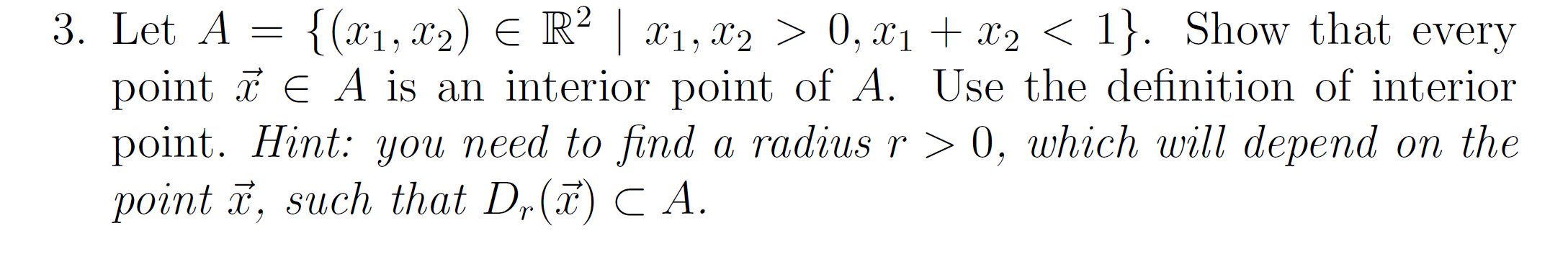# Question Solved1 Answerplease use graph to prove that d_r(x) is subset of set A. and set A is a open set that is a triangle, not a quarter circle. 3. Let A = {(x1, x2) E R² | x1, x2 > 0, x1 + x2 < 1}. Show that every point T E A is an interior point of A. Use the definition of interior point. Hint: you need to find a radius r > 0, which will depend on the point ž, such that D,(ã) CA.please use graph to prove that d_r(x) is subset of set A. and set A is a open set that is a triangle, not a quarter circle.

Transcribed Image Text: 3. Let A = {(x1, x2) E R² | x1, x2 > 0, x1 + x2 < 1}. Show that every point T E A is an interior point of A. Use the definition of interior point. Hint: you need to find a radius r > 0, which will depend on the point ž, such that D,(ã) CA.
More
Transcribed Image Text: 3. Let A = {(x1, x2) E R² | x1, x2 > 0, x1 + x2 < 1}. Show that every point T E A is an interior point of A. Use the definition of interior point. Hint: you need to find a radius r > 0, which will depend on the point ž, such that D,(ã) CA.